## Thursday, January 31, 2019

### Unweighted & weighted square-change-parsimony vs. ML ancestral state estimation

A R-sig-phylo member today wrote:

“I want to get squared change parsimony ancestral state reconstructions for a matrix into R.

I have tried the `asr_max_parsimony()` function from castor, but the results are not the same as in Mesquite for example. To take an example, a two-taxon clade with a unique state for these taxa optimized as having a different state (shared by near relatives) at the ancestral node. This doesn't happen in Mesquite and is not what I want.

If there were some way to get the results from Mesquite into R that would also be OK, but I cannot figure out the node numbering system in Mesquite, to match them up with nodes as numbered by ape.”

My response to this is that square-change parsimony ancestral states (defined as the states that minimize the unweighted sum of square changes implied across all the edges of the tree) are the same as the ML ancestral states in which we set all edge lengths to the same (non-zero) value.

To demonstrate this, we can easily write our own simple square-change-parsimony function as follows:

``````square.change.parsimony<-function(tree,x,...){
if(hasArg(maxit)) maxit<-list(...)\$maxit
else maxit<-2000
if(hasArg(trace)) trace<-list(...)\$trace
else trace<-FALSE
if(hasArg(init)) init<-list(...)\$init
else init<-NULL
SSCH<-function(a,x,phy,trace){
A<-matrix(c(x[phy\$tip.label],
a[as.character(1:phy\$Nnode+Ntip(phy))])[phy\$edge],
nrow(phy\$edge),2)
SS<-sum((A[,2]-A[,1])^2)
if(trace) cat(paste("Sum of Squares :",round(SS,8),"\n"))
SS
}
if(is.null(init)) init<-fastAnc(tree,x)
fit<-optim(init,SSCH,x=x,phy=tree,trace=trace,
control=list(maxit=maxit))
object<-list(ace=unclass(fit\$par),sum.of.squares=fit\$value,
counts=fit\$counts,convergence=fit\$convergence,
message=fit\$message)
object
}
``````

Now we can compare it to ML values obtained (say) using `fastAnc`, but in which we first set all edge lengths of the tree to 1.0.

``````## simulate some data
library(phytools)
tree<-pbtree(n=26,tip.label=LETTERS)
x<-fastBM(tree)

## our square change parsimony states:
fit<-square.change.parsimony(tree,x,maxit=1e8) ## we have to set maxit to be quite high
fit
``````
``````## \$ace
##          27          28          29          30          31          32
## -0.58984343 -0.67455123 -0.06632310 -0.67313617 -0.05265349  0.86007564
##          33          34          35          36          37          38
## -1.95745914  1.22430357  1.36003054  0.62492090 -0.23894155  2.40076293
##          39          40          41          42          43          44
##  2.69448380  2.78748896 -1.39183963 -1.36220597 -2.07969284 -0.47162174
##          45          46          47          48          49          50
## -1.03879642 -1.19275392 -1.09041472 -1.08289504 -1.00470802 -1.02584958
##          51
## -1.32344518
##
## \$sum.of.squares
##  15.00555
##
## \$counts
##     7590       NA
##
## \$convergence
##  0
##
## \$message
## NULL
``````
``````## our ML states with all edges to 1.0:
utree<-tree
utree\$edge.length<-rep(1,nrow(tree\$edge))
sch<-fastAnc(utree,x)
sch
``````
``````## Ancestral character estimates using fastAnc:
##        27        28        29        30        31        32        33
## -0.491222 -0.597435  0.017300 -0.640381 -0.019996  0.868693 -1.918447
##        34        35        36        37        38        39        40
##  1.289716  1.417875  0.649351 -0.236074  2.433972  2.716463  2.802910
##        41        42        43        44        45        46        47
## -1.318385 -1.319005 -2.038713 -0.385008 -0.987479 -1.155175 -1.064055
##        48        49        50        51
## -1.050179 -0.978863 -0.983751 -1.297779
``````
``````## compare them
plot(fit\$ace,sch,pch=21,bg="grey",cex=2,xlab="square change parsimony",
ylab="ML on tree with edge lengths to 1.0")
``````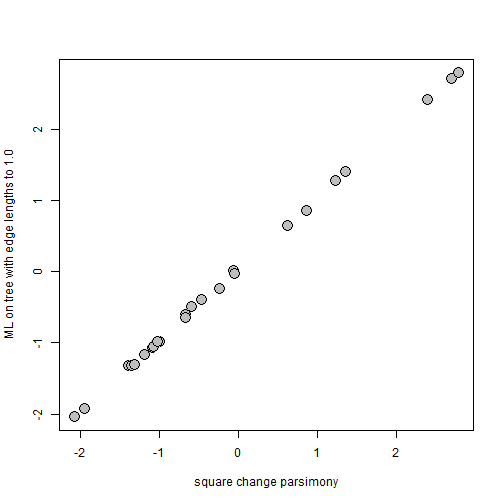If they're different, it may just be because we haven't converged on the genuine minimum sum of squares solution. We could run our optimization longer, change our tolerance, or (best of all) start with a superior solution. Let's try the lattermost of these options:

``````fit2<-square.change.parsimony(tree,x,maxit=1e8,init=sch)
fit
``````
``````## \$ace
##          27          28          29          30          31          32
## -0.58984343 -0.67455123 -0.06632310 -0.67313617 -0.05265349  0.86007564
##          33          34          35          36          37          38
## -1.95745914  1.22430357  1.36003054  0.62492090 -0.23894155  2.40076293
##          39          40          41          42          43          44
##  2.69448380  2.78748896 -1.39183963 -1.36220597 -2.07969284 -0.47162174
##          45          46          47          48          49          50
## -1.03879642 -1.19275392 -1.09041472 -1.08289504 -1.00470802 -1.02584958
##          51
## -1.32344518
##
## \$sum.of.squares
##  15.00555
##
## \$counts
##     7590       NA
##
## \$convergence
##  0
##
## \$message
## NULL
``````
``````plot(fit2\$ace,sch,pch=21,bg="grey",cex=2,xlab="square change parsimony",
ylab="ML on tree with edge lengths to 1.0")
``````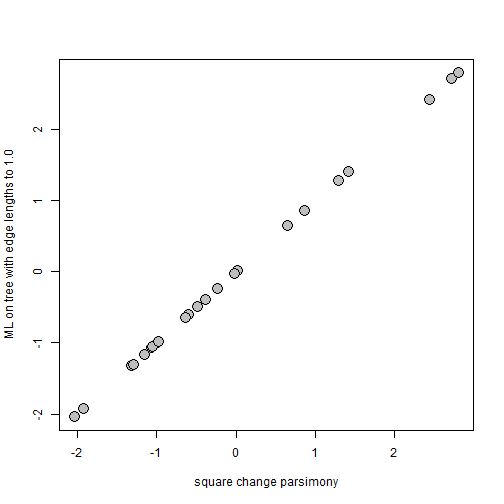Now, as a point of reference, let's compare to our standard ML estimates:

``````plot(fastAnc(tree,x),fit\$ace,pch=21,bg="grey",cex=2,xlab="regular ML",
ylab="square change parsimony")
``````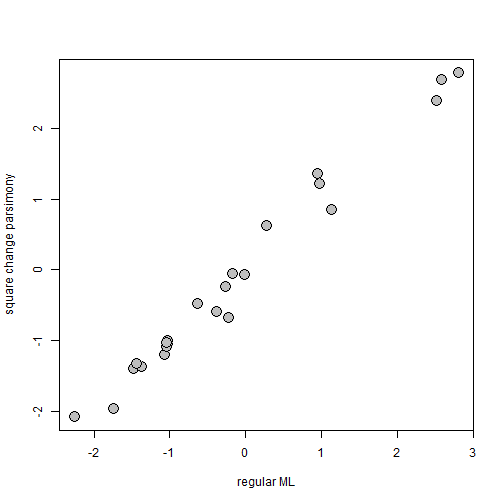So, in other words, there is no need to for a special square-change-parsimony function as you should be able to just use `ace` or `fastAnc`, but with all edges set to unit length; and this also tells us something important about square-change-parsimony - that (in its unweighted form) it assumes that the amount of change between a reconstructed ancestral value and any tip depends only on the number of edges separating them - and not at all on the length of those edges.

Finally, if we adapt our previous function such that we divide each squared change by the corresponding edge length before summing them:

``````weighted.square.change.parsimony<-function(tree,x,...){
if(hasArg(maxit)) maxit<-list(...)\$maxit
else maxit<-2000
if(hasArg(trace)) trace<-list(...)\$trace
else trace<-FALSE
if(hasArg(init)) init<-list(...)\$init
else init<-NULL
SSCH<-function(a,x,phy,trace){
A<-matrix(c(x[phy\$tip.label],
a[as.character(1:phy\$Nnode+Ntip(phy))])[phy\$edge],
nrow(phy\$edge),2)
SS<-sum((A[,2]-A[,1])^2/phy\$edge.length)
if(trace) cat(paste("Sum of Squares :",round(SS,8),"\n"))
SS
}
if(is.null(init)) init<-fastAnc(tree,x)
fit<-optim(init,SSCH,x=x,phy=tree,trace=trace,
control=list(maxit=maxit))
object<-list(ace=unclass(fit\$par),sum.of.squares=fit\$value,
counts=fit\$counts,convergence=fit\$convergence,
message=fit\$message)
object
}
``````

an analysis that has been called weighted square-change-parsimony, it turns out that this is exactly the same as regular ML:

``````fit3<-weighted.square.change.parsimony(tree,x)
plot(fit3\$ace,fastAnc(tree,x),pch=21,bg="grey",cex=2,
xlab="weighted square change parsimony",ylab="ML")
``````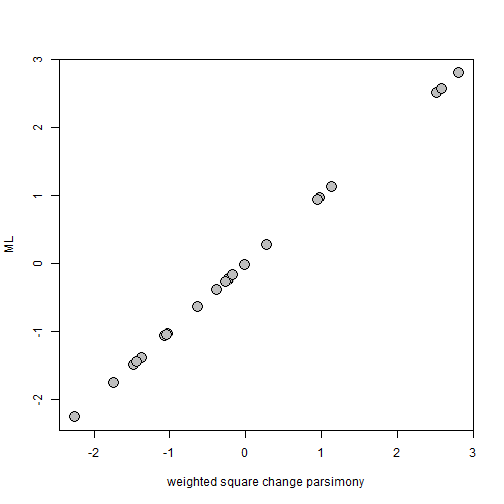That's it.

## Saturday, January 19, 2019

### MCCR test now in phytools

Yesterday I posted about implementing Pybus & Harvey's (2000) MCCR test. This used to be in Dan Rabosky's laser, which is no longer available (except in archived versions) on CRAN. The method is quite simple as it merely uses Monte Carlo simulation to generate a null distribution of the γ-statistic for incompletely sampled phylogenies. I have now added this function (and a couple of associated methods) to phytools. This update can be obtained by installing the latest version of phytools from GitHub using devtools.

Here's a quick demo of how the function works:

``````library(phytools)
packageVersion("phytools")
``````
``````##  '0.6.66'
``````
``````tree<-pbtree(n=600,scale=100)
ltt(tree,show.tree=TRUE,lwd=3)
``````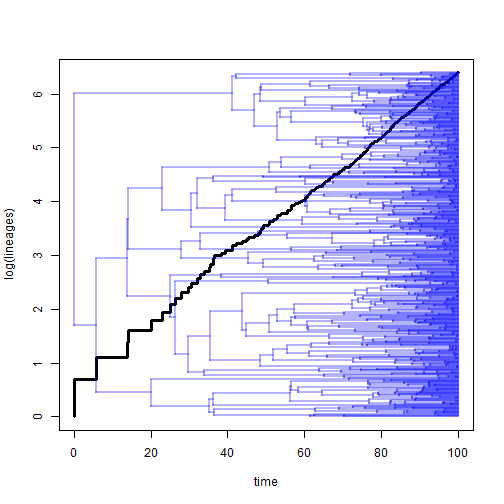``````## Object of class "ltt" containing:
##
## (1) A phylogenetic tree with 600 tips and 599 internal nodes.
##
## (2) Vectors containing the number of lineages (ltt) and branching times (times) on the tree.
##
## (3) A value for Pybus & Harvey's "gamma" statistic of -0.1158, p-value = 0.9078.
``````
``````## subsample the tree to introduce a downward bias in gamma:
incomplete<-drop.tip(tree,sample(tree\$tip.label,400))
ltt(incomplete,show.tree=TRUE,lwd=3)
``````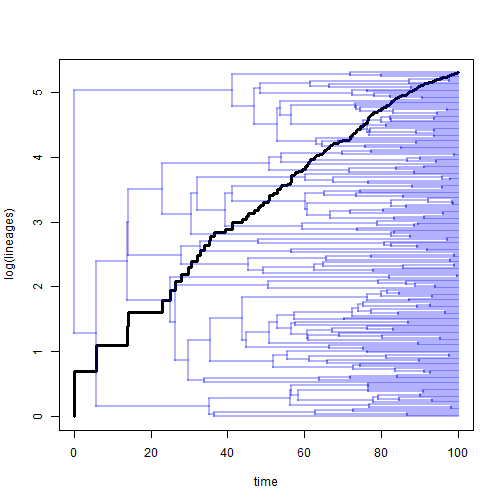``````## Object of class "ltt" containing:
##
## (1) A phylogenetic tree with 200 tips and 199 internal nodes.
##
## (2) Vectors containing the number of lineages (ltt) and branching times (times) on the tree.
##
## (3) A value for Pybus & Harvey's "gamma" statistic of -4.4687, p-value = 0.
``````
``````## MCCR test to take into account incomplete sampling:
object<-ltt(incomplete,plot=FALSE)
mccr.result<-mccr(object,rho=200/600,nsim=500)
mccr.result
``````
``````## Object of class "mccr" consisting of:
##
## (1) A value for Pybus & Harvey's "gamma" statistic of -4.4687.
##
## (2) A two-tailed p-value from the MCCR test of 0.92.
##
## (3) A simulated null-distribution of gamma from 500 simulations.
``````
``````plot(mccr.result)
``````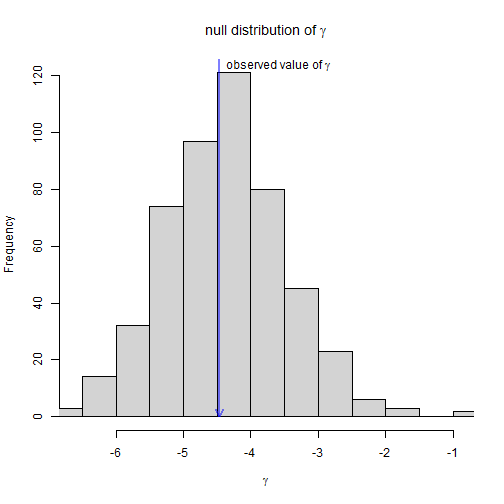We can also do a small experiment to show that the MCCR test has the correct type I error rate. Here I'm going to simulate five hundred 150 taxon trees, each of which I proceed to randomly subsample to include only 100 taxa. I'll then compute γ & a P-value for the null hypothesis test of γ equal to zero on the original tree; the same things on the subsampled tree; and finally a P-value based on the MCCR method:

``````foo<-function(){
full<-pbtree(n=150)
full.ltt<-ltt(full,plot=FALSE)
incomplete<-drop.tip(full,sample(full\$tip.label,50))
incomplete.ltt<-ltt(incomplete,plot=FALSE)
setNames(c(full.ltt\$gamma,full.ltt\$p,
incomplete.ltt\$gamma,incomplete.ltt\$p,
mccr(incomplete.ltt,rho=100/150,nsim=200)\$'P(two-tailed)'),
c("full-gamma","full-P(gamma)","inc-gamma",
"inc-P(gamma)","P(mccr)"))
}
sim.Result<-t(replicate(500,foo()))
bb<-seq(0,1,by=0.05)
par(mfrow=c(3,1))
obj<-hist(sim.Result[,2],bb,plot=FALSE)
obj\$counts<-obj\$counts/nrow(sim.Result)
plot(obj,ylab="relative frequency",col="grey",ylim=c(0,0.4),xlab="P-value",main="")
lines(c(0,1),rep(0.05,2),col="blue",lty="dotted")
mtext(text="a) P-value distribution on complete trees",adj=0,line=1,
cex=1)
obj<-hist(sim.Result[,4],bb,plot=FALSE)
obj\$counts<-obj\$counts/nrow(sim.Result)
plot(obj,ylab="relative frequency",col="grey",ylim=c(0,0.4),xlab="P-value",main="")
lines(c(0,1),rep(0.05,2),col="blue",lty="dotted")
mtext(text="b) P-value distribution on incompletely sampled trees",adj=0,line=1,
cex=1)
obj<-hist(sim.Result[,5],bb,plot=FALSE)
obj\$counts<-obj\$counts/nrow(sim.Result)
plot(obj,ylab="relative frequency",col="grey",ylim=c(0,0.4),xlab="P-value",main="")
lines(c(0,1),rep(0.05,2),col="blue",lty="dotted")
mtext(text="c) P-value distribution on incompletely sampled trees using MCCR test",
``````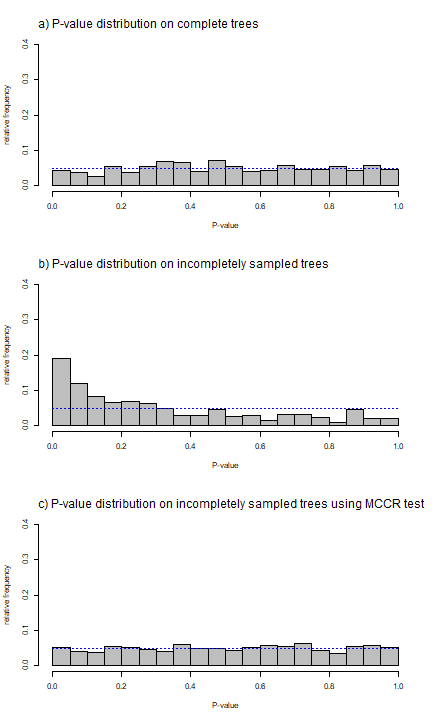We can see that the parametric test has good type I error on completely sampled tree, but elevated error on incompletely sampled trees. The MCCR test recovers this good type I error of the parametric test when our taxon sampling is incomplete. We can also compute the type I errors directly as follows:

``````typeI<-setNames(c(mean(sim.Result[,2]<=0.05),
mean(sim.Result[,4]<=0.05),mean(sim.Result[,5]<=0.05)),
c("complete","incomplete","MCCR"))
typeI
``````
``````##   complete incomplete       MCCR
##      0.044      0.190      0.052
``````

Neat.

## Friday, January 18, 2019

### MCCR test for Pybus & Harvey's γ on incompletely sampled trees using phytools

The MCCR test for Pybus & Harvey's (2000) γ statistic from incompletely sampled phylogenies used to be implemented in the no-longer-available-on-CRAN laser package of Dan Rabosky. The method is pretty simple, though, & since we use it in teaching I figured it would be easy enough to add to phytools.

Here is a function to do the test, as well as `print` & `plot` methods for the resultant object:

``````mccr<-function(obj,rho=1,nsim=100,plot=TRUE){
N<-round(Ntip(obj\$tree)/rho)
tt<-pbtree(n=N,nsim=nsim)
foo<-function(x,m) drop.tip(x,sample(x\$tip.label,m))
tt<-lapply(tt,foo,m=N-Ntip(obj\$tree))
g<-sapply(tt,function(x) ltt(x,plot=FALSE)\$gamma)
P<-if(obj\$gamma>median(g)) 2*mean(g>=obj\$gamma) else 2*mean(g<=obj\$gamma)
result<-list(gamma=obj\$gamma,"P(two-tailed)"=P,null.gamma=g)
class(result)<-"mccr"
result
}

print.mccr<-function(x,digits=4,...){
cat("Object of class \"mccr\" consisting of:\n\n")
cat(paste("(1) A value for Pybus & Harvey's \"gamma\"",
" statistic of ",round(x\$gamma,digits),".\n\n",sep=""))
cat(paste("(2) A two-tailed p-value from the MCCR test of ",
round(x\$'P(two-tailed)',digits),".\n\n", sep = ""))
cat(paste("(3) A simulated null-distribution of gamma from ",
length(x\$null.gamma)," simulations.\n\n",sep=""))
}

plot.mccr<-function(x,...){
hist(x\$null.gamma,breaks=min(c(max(12,round(length(x\$null.gamma)/10)),20)),
xlim=range(c(x\$gamma,x\$null.gamma)),
main=expression(paste("null distribution of ",
gamma)),xlab=expression(gamma),col="lightgrey")
arrows(x0=x\$gamma,y0=par()\$usr,y1=0,length=0.12,
col=make.transparent("blue",0.5),lwd=2)
text(x\$gamma,0.98*par()\$usr,
expression(paste("observed value of ",gamma)),
pos=if(x\$gamma>mean(x\$null.gamma)) 2 else 4)
}
``````

Now let's see how to use it. First, I'll run it on the completely sampled tree:

``````## pure birth tree with complete sampling:
pb<-pbtree(n=100)
lineages<-ltt(pb)
``````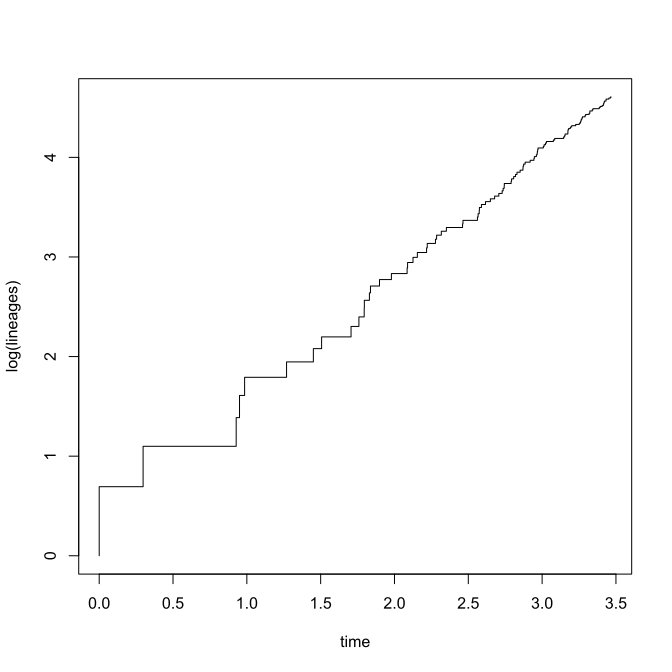``````lineages
``````
``````## Object of class "ltt" containing:
##
## (1) A phylogenetic tree with 100 tips and 99 internal nodes.
##
## (2) Vectors containing the number of lineages (ltt) and branching times (times) on the tree.
##
## (3) A value for Pybus & Harvey's "gamma" statistic of -0.2688, p-value = 0.7881.
``````
``````## MCCR test assuming complete sampling:
object<-mccr(lineages,rho=1,nsim=200)
object
``````
``````## Object of class "mccr" consisting of:
##
## (1) A value for Pybus & Harvey's "gamma" statistic of -0.2688.
##
## (2) A two-tailed p-value from the MCCR test of 0.67.
##
## (3) A simulated null-distribution of gamma from 200 simulations.
``````
``````plot(object)
``````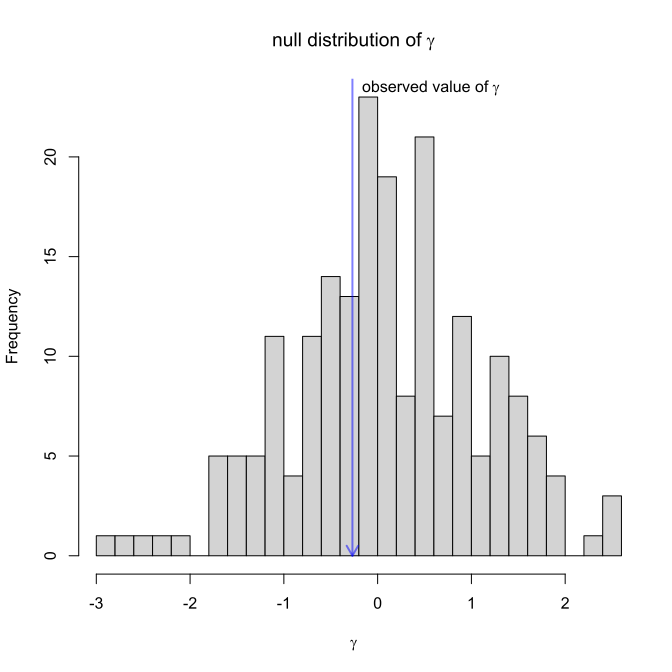A neat thing to show is that the MCCR test (based on simulation) will give a P-value that converges on the P-value from the parametric test if you run enough simulations. Let's see that:

``````object<-mccr(lineages,rho=1,nsim=10000)
lineages
``````
``````## Object of class "ltt" containing:
##
## (1) A phylogenetic tree with 100 tips and 99 internal nodes.
##
## (2) Vectors containing the number of lineages (ltt) and branching times (times) on the tree.
##
## (3) A value for Pybus & Harvey's "gamma" statistic of -0.2688, p-value = 0.7881.
``````
``````object
``````
``````## Object of class "mccr" consisting of:
##
## (1) A value for Pybus & Harvey's "gamma" statistic of -0.2688.
##
## (2) A two-tailed p-value from the MCCR test of 0.7942.
##
## (3) A simulated null-distribution of gamma from 10000 simulations.
``````

Next, we can simulate incomplete sampling, see the effect on the P-value from `ltt`, and then compare that to a P-value obtained from the MCCR test:

``````incomplete.pb<-drop.tip(pb,sample(pb\$tip.label,round(0.5*Ntip(pb))))
lineages<-ltt(incomplete.pb,plot=FALSE)
lineages
``````
``````## Object of class "ltt" containing:
##
## (1) A phylogenetic tree with 50 tips and 49 internal nodes.
##
## (2) Vectors containing the number of lineages (ltt) and branching times (times) on the tree.
##
## (3) A value for Pybus & Harvey's "gamma" statistic of -0.9876, p-value = 0.3234.
``````
``````object<-mccr(lineages,rho=0.5,nsim=200)
object
``````
``````## Object of class "mccr" consisting of:
##
## (1) A value for Pybus & Harvey's "gamma" statistic of -0.9876.
##
## (2) A two-tailed p-value from the MCCR test of 0.76.
##
## (3) A simulated null-distribution of gamma from 200 simulations.
``````

Finally, for fun we can simulate a tree with a shape that should correspond to a positive value of γ. Here, I'll do it using the phytools internal function `ebTree`. We can then subsample the tree to simulate incomplete sampling.

``````eb<-phytools:::ebTree(pbtree(n=200,scale=1),-1.2)
ltt(eb)
``````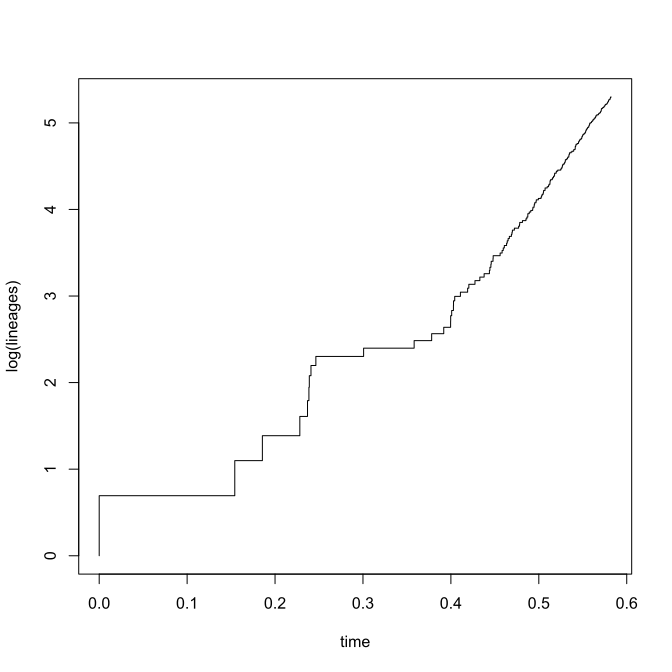``````## Object of class "ltt" containing:
##
## (1) A phylogenetic tree with 200 tips and 199 internal nodes.
##
## (2) Vectors containing the number of lineages (ltt) and branching times (times) on the tree.
##
## (3) A value for Pybus & Harvey's "gamma" statistic of 2.0067, p-value = 0.0448.
``````
``````incomplete.eb<-drop.tip(eb,sample(eb\$tip.label,round(0.5*Ntip(eb))))
ltt(incomplete.eb)
``````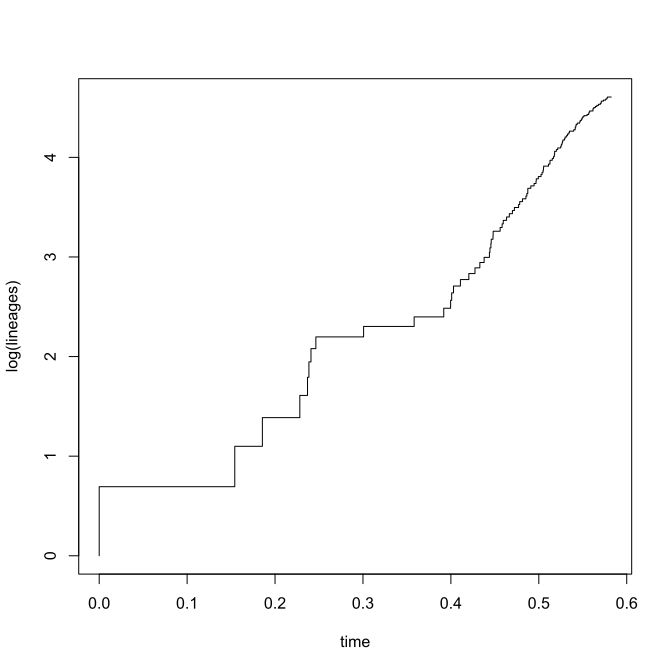``````## Object of class "ltt" containing:
##
## (1) A phylogenetic tree with 100 tips and 99 internal nodes.
##
## (2) Vectors containing the number of lineages (ltt) and branching times (times) on the tree.
##
## (3) A value for Pybus & Harvey's "gamma" statistic of -0.2614, p-value = 0.7938.
``````
``````object<-mccr(ltt(incomplete.eb,plot=FALSE),rho=0.5,nsim=200)
object
``````
``````## Object of class "mccr" consisting of:
##
## (1) A value for Pybus & Harvey's "gamma" statistic of -0.2614.
##
## (2) A two-tailed p-value from the MCCR test of 0.06.
##
## (3) A simulated null-distribution of gamma from 200 simulations.
``````
``````plot(object)
``````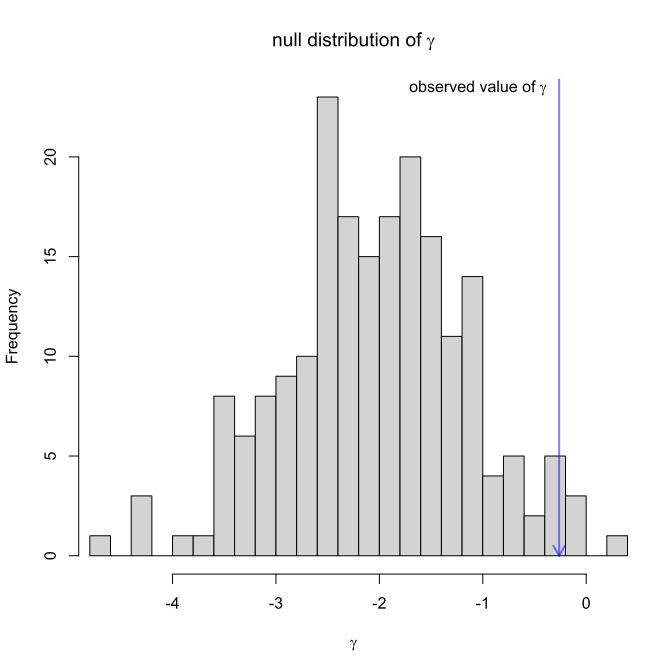Neat.

This is not yet in phytools, but I will add documentation & put it in a future release.